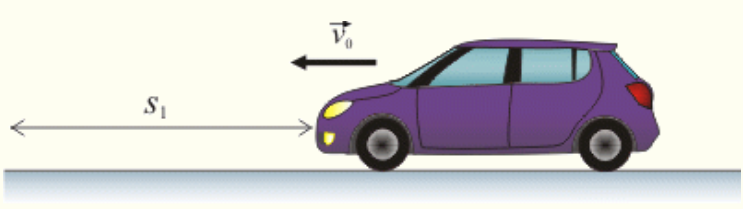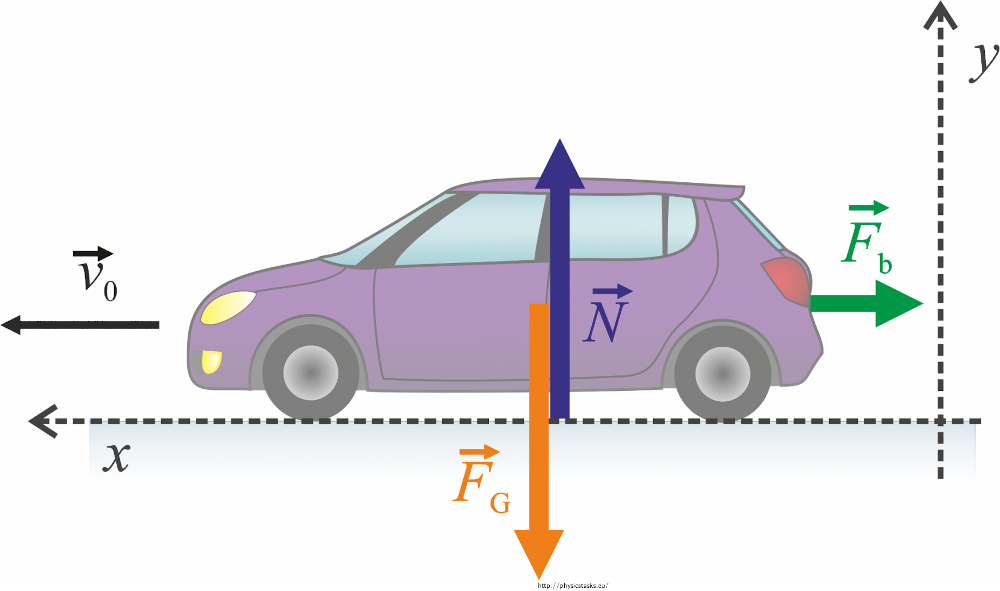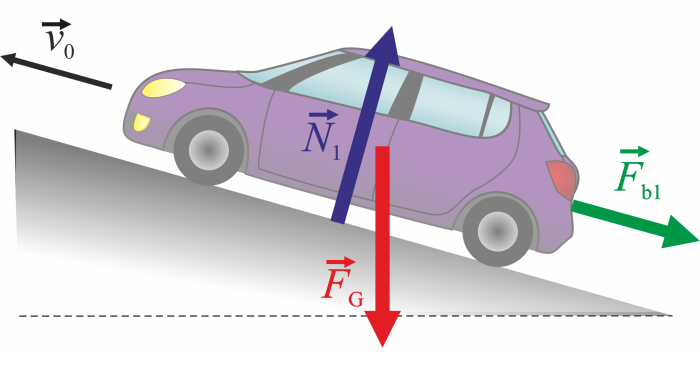# Braking car problem

When the brake pedal of a car moving 80 km/h on a straight asphalt road is fully pressed, the car can stop in 50 m. How long will its braking distance be on an identical road which forms a 5° angle with the horizontal plane? Consider both the case when it brakes downhill and when it brakes uphill. Assume that at the time t = 0 s the coordinate is x = 0 m. The wheels do not slip. Neglect the air resistance.Please enter the following data to calculate the previous equation

Enter Force breaking

Enter mass

Equation 3 result is

Comment:

Enter initial speed

Enter time

Enter Force breaking

Enter mass

Equation 5 result is

Comment:

-

Enter mass

Enter time

Enter Force breaking

Enter initial speed

equation 7 result is

Comment:

Enter mass

Enter time

Enter Force breaking

Enter initial speed

equation 8 result is

Comment:

### Equation 10 from problem 30

 Enter m Enter v0 Enter Ss Equation 10 result is Fb Comment 10:

Note: The kinetic energy of a car at the beginning of its brakins is qual to the work done by the braking force: W=Ek

### Fb=f*N=f*m*g

Fb..frictional braking force

f..coeficient of static friction

N..force wich the car acts upon the road

m..the mass of the object

g..the acceleration of gravity

### Equation 11 from problem 30

 Enter f Enter m Enter g Equation 11 result is Fb Comment 11:

The coefficient of friction f will be the sabe in both scenarios

We substitute for Fb from relation (10)

### Equation 12 from problem 30

 Enter g Enter v0 Enter Ss Equation 12 result is F Comment 12:

#### Forces acting upon a car moving uphill:

Fg.. gravitational force

N1..normal force with which the road upon the car

Fb1..braking force### Fb1 + Fg + N1 = m * a1

We rewrite the equation using scalars and choose a coordinate system such that the x axis is parallel to the movement of the car.

The y axis is perpendicular to the x axis. We split the gravitational force into two components in the directions of the axes:

Enter m

Enter s

Enter v

Enter α

Equation 15 result is Fb1

We express force N from relation (14) and substitute for it

We substitute for f from relation to (12)

Comment:

Enter Fb

Enter g

Enter m

Enter α

Equation 16 result is a1

We evaluate acceleration from equation (13)

Comment:

Enter m

Enter g

Enter Fb

Enter t

Enter α

Equation 18 result is v1

If we rewrite equation (17), we obtain the dependency of the velocity of the car velocity on time

By integrating relation (18) we obtain the dependency of the coordinate time

Comment:

Enter m

Enter t

Enter Fb

Enter α

Equation 20 result is x1

If we rewrite equation (19), we obtain the dependency of the dependency of the coordinate of the car on time

We calculate the time of stopping t1 by substituting zero for velocity into relation (18)

Comment:

Enter Fb

Enter m

Enter g

Enter v

Enter α

Equation 21 result is Ts

We substiyute the time t1 into relation (20) and calculate the total distance Ss travelled by the car

Comment:

Enter g

Enter s

Enter v

Enter α

equation 22 result is Ss

We substitute for Fb into relation (15)

Comment:

Enter m

Enter g

Enter v

Enter Ss

Equation 25 result is Fb

Comment25:

.

Enter Ts2

Enter Fb2

Enter m

Enter g

Enter α

Equation 26 result is a2

Comment:

.

Enter Fb2

Enter m

Enter g

Enter α

Enter Ts2

Equation 31 result is v0

Comment:

.

Enter Fb2

Enter m

Enter g

Enter v0

Enter α

Equation 32 result is ts2

Comment:

.

Enter m

Equation 33 result is ss2

Comment: